# Any large square for general k

To warm up, let’s start with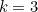. We want to prove that the numbers within the large square that’s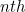from the left ad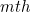from the top sum to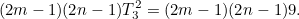First look at the large square that’sfrom the left and lies in the first row of large squares. As we already noted above, the two numbers in the top row of this white square are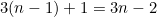and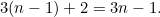By some very similar reasoning we can work out that the top two numbers in the large square that’sfrom the left andfrom the top are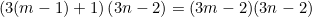and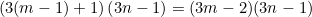Similarly, the bottom two numbers in thewhite square from the left in therow of white squares are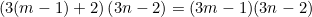and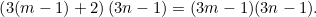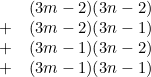which we can rewrite as the product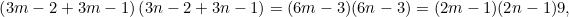which is exactly what we wanted.

We now move on to prove the result for any positive integer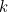. We want to show that the sum of numbers in the square that’sfrom the left andfrom the top is equal to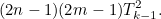Let’s call the square in question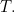Using similar reasoning as above, you can see that the numbers in the first row of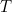are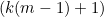times the numbers in the first row of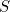. This means that the sum of the first row of numbers inis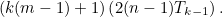Similarly, the sum of the second row of numbers inis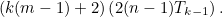We can continue in this vein until we come to the sum of the last row of numbers inwhich is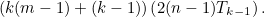Adding up these sums of the rows ofgives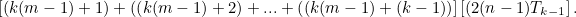(1)

Similarly to what we did above, we can re-write this expression as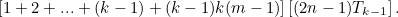Using the formula for the sum of the first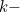integers, this becomes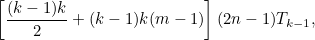which is equal to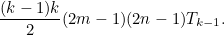And since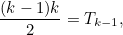the sum of the numbers inis equal to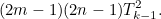This is what we wanted to show.